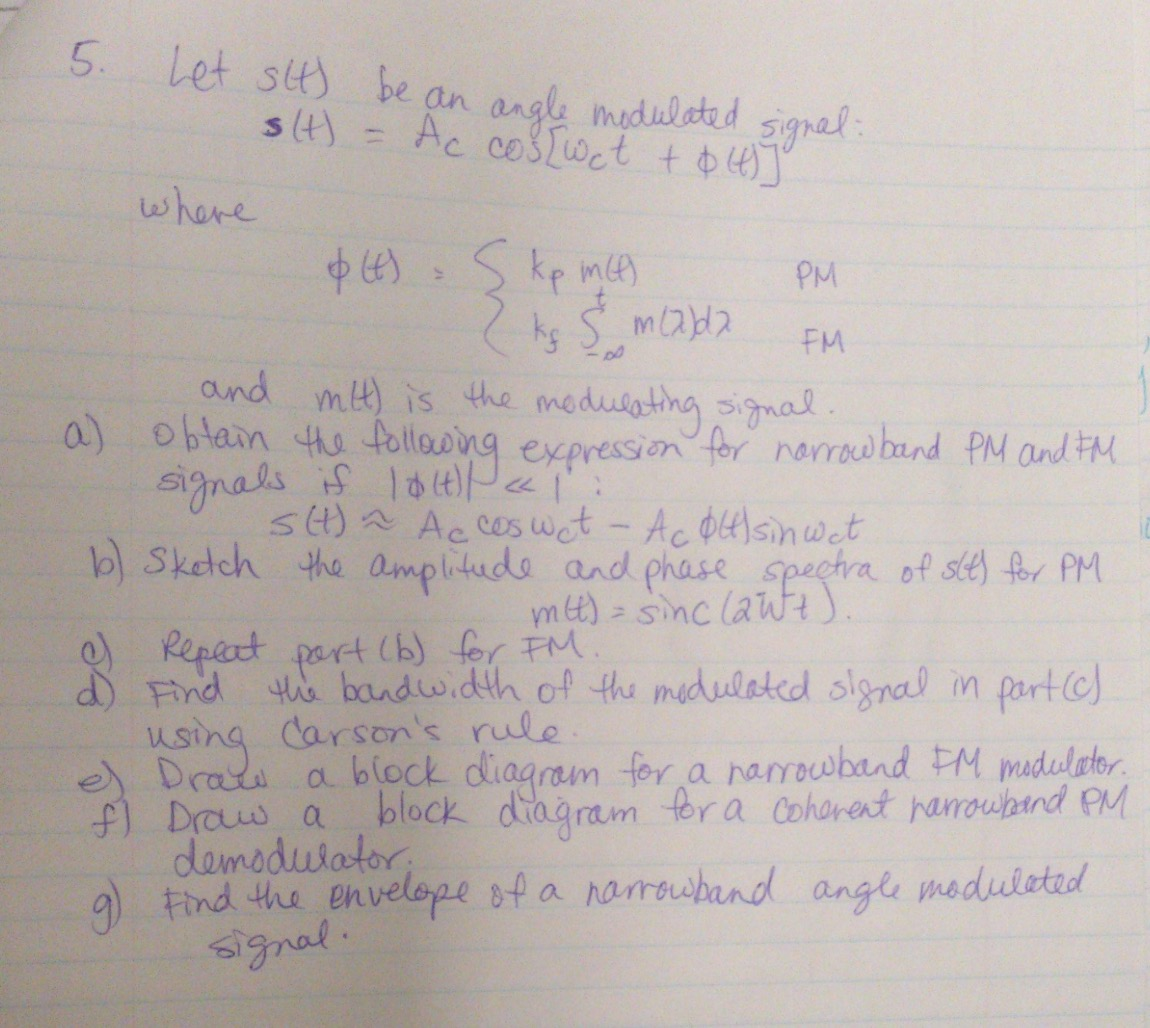## Let s(t) be an angle modulated signal: s(t) = A_c cos[w_c t + (t)] Where (t) = {k_p m(t) PM k_f...Solved